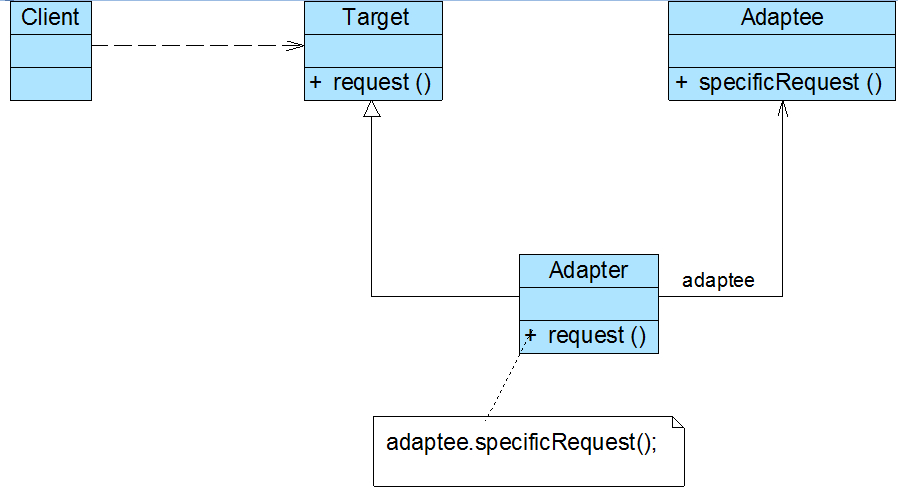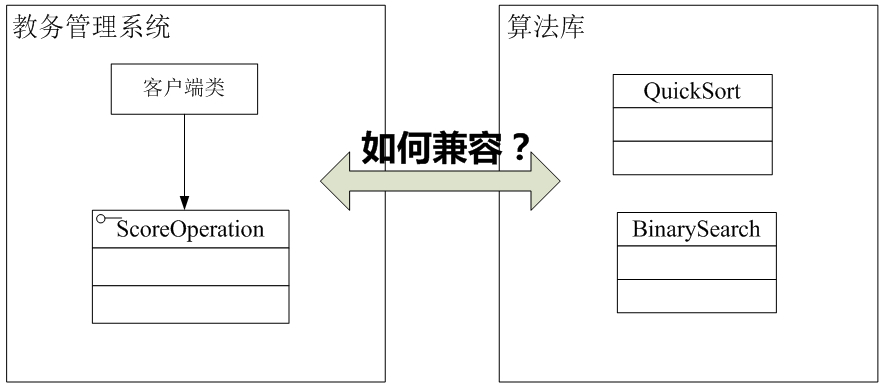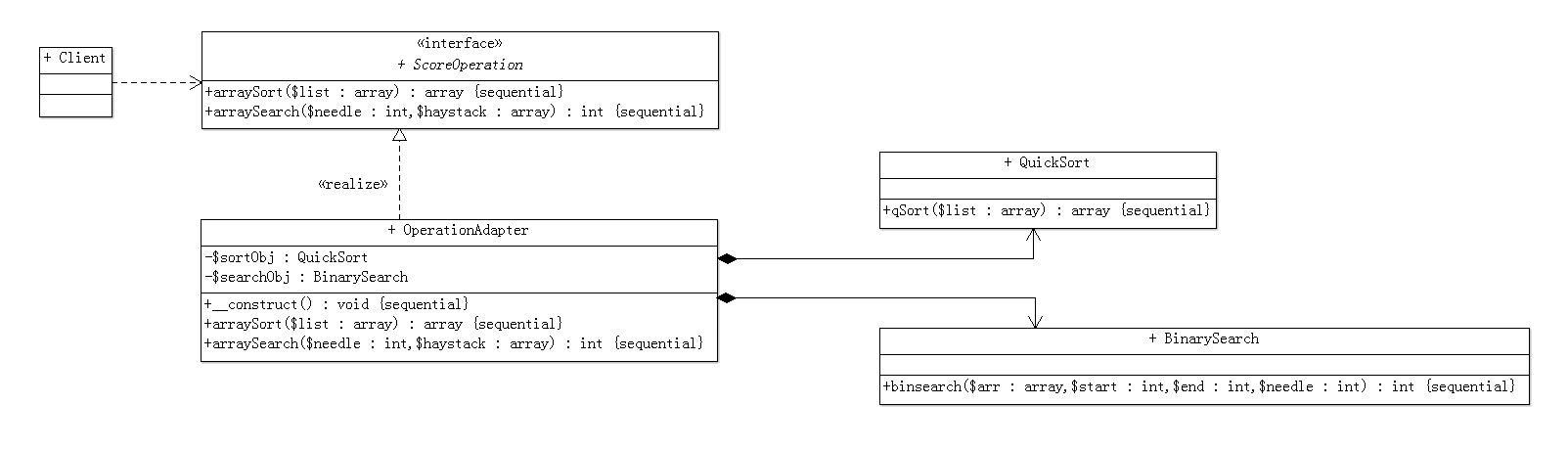### 0. 前言### 1. 模式定义

• 适配器模式可以将一个类的接口和另一个类的接口匹配起来，而无须修改原来的适配者接口和抽象目标类接口。
• 在适配器模式定义中所提及的接口是指广义的接口，它可以表示一个方法或者方法的集合。
• 在适配器模式中，我们通过增加一个新的适配器类来解决接口不兼容的问题，使得原本没有任何关系的类可以协同工作。根据适配器类与适配者类的关系不同，适配器模式可分为对象适配器和类适配器两种，在对象适配器模式中，适配器与适配者之间是关联关系；在类适配器模式中，适配器与适配者之间是继承（或实现）关系。在实际开发中，对象适配器的使用频率更高。

### 2. 对象适配器模式结构

• Target目标抽象类

• 目标抽象类定义客户所需接口，可以是一个抽象类或接口，也可以是具体类。
• Adaptee适配者类

• 适配者即被适配的角色，它定义了一个已经存在的接口，这个接口需要适配，适配者类一般是一个具体的类，包含了客户希望使用的业务方法，在某些情况下可能没有适配者类的源代码。
• Adapter适配器类### 实例说明

Sunny软件公司在很久以前曾开发了一个算法库，里面包含了一些常用的算法，例如排序算法和查找算法，在进行各类软件开发时经常需要重用该算法库中的算法。在为某学校开发教务管理系统时，开发人员发现需要对学生成绩进行排序和查找，该系统的设计人员已经开发了一个成绩操作接口ScoreOperation，在该接口中声明了排序方法arraySort(array $list): array和查找方法arraySearch(int$needle, array $haystack): int，为了提高排序和查找的效率，开发人员决定重用算法库中的快速排序算法类QuickSort和二分查找算法类BinarySearch，其中QuickSort的qSort(array$list): array方法实现了快速排序，BinarySearch的binsearch(array $arr, int$start, int $end, int$needle): int方法实现了二分查找。

Sunny软件公司开发人员面对这个没有源码的算法库，遇到一个幸福而又烦恼的问题：如何在既不修改现有接口又不需要任何算法库代码的基础上能够实现算法库的重用？### 实例代码

• ScoreOperation：目标抽象类
/**
* 抽象成绩操作类：目标抽象类
*
* @author     <dendi875@163.com>
* @createDate 2018-06-25
*/

interface ScoreOperation
{
public function arraySort(array $list): array; public function arraySearch(int$needle, array $haystack): int; } • QuickSort、BinarySearch： 适配者类 /** * 快速排序类：适配者 * * @author <dendi875@163.com> * @createDate 2018-06-25 * @copyright Copyright (c) 2018 dendi875@163.com */ class QuickSort { public function qSort(array$list): array
{
if (count($list) <= 1) { return$list;
}

$baseValue =$list;

$leftArray =$rightArray = [];

array_shift($list); foreach ($list as $value) { if ($value < $baseValue) {$leftArray[] = $value; } else {$rightArray[] = $value; } }$leftArray = $this->qSort($leftArray);
$rightArray =$this->qSort($rightArray); return array_merge($leftArray, array($baseValue),$rightArray);
}
}

/**
* 二分查找类：适配者
*
* @author     <dendi875@163.com>
* @createDate 2018-06-25
*/

class BinarySearch
{
public function binsearch(array $arr, int$start, int $end, int$needle): int
{
if ($start >$end) {
return -1;
}

$mid = ceil(($end + $start) / 2); if ($arr[$mid] >$needle) {
return $this->binsearch($arr, $start,$end - 1, $needle); } else if ($arr[$mid] <$needle) {
return $this->binsearch($arr, $start + 1,$end, $needle); } else { return$mid;
}
}
}
• OperationAdapter： 适配器类
/**
* 操作适配器：适配器类
*
* @author     <dendi875@163.com>
* @createDate 2018-06-25
*/

{
private $sortObj; private$searchObj;

public function __construct()
{
$this->sortObj = new QuickSort();$this->searchObj = new BinarySearch();
}

public function arraySort(array $list): array { return$this->sortObj->qSort($list); } public function arraySearch(int$needle, array $haystack): int { return$this->searchObj->binsearch($haystack, 0, count($haystack) - 1, $needle); } } • 客户端测试代码 /* 定义成绩数组 */$scores = [90, 84, 76, 50, 69, 90, 91, 88, 96];
$operation = new OperationAdapter();$result = $operation->arraySort($scores);
echo "成绩排序结果：".implode(',', $result); //output：成绩排序结果：50,69,76,84,88,90,90,91,96$key1 = $operation->arraySearch(90,$result);
$key2 =$operation->arraySearch(84, $result);$key3 = $operation->arraySearch(100,$result);
echo "查找成绩为90的索引位置：".$key1; //output：查找成绩为90的索引位置：5 echo "查找成绩84的索引位置：".$key2;   //output：查查找成绩84的索引位置：3
echo "查找成绩100的索引位置：".\$key3;  //output：查找成绩100的索引位置：-1

### 4. UML类图### 5. 适配器模式的优点

(1)将目标类和适配者类解耦，通过引入一个适配器类来重用现有的适配者类，无须修改原有结构。

(2)增加了类的透明性和复用性，将具体的业务实现过程封装在适配者类中，对于客户端类而言是透明的，而且提高了适配者的复用性，同一个适配者类可以在多个不同的系统中复用。

(3)一个对象适配器可以把多个不同的适配者适配到同一个目标。

### 6. 适配器模式的缺点

(1)PHP不支持多继承，一次最多只能适配一个适配者类，不能同时适配多个适配者；但可以通过Trait来减少单继承的限制。

(2)适配者类不能为最终类，如在PHP中不能为final类；

### 7.适配器模式适用场景

(1)系统需要使用一些现有的类，而这些类的接口（如方法名）不符合系统的需要，甚至没有这些类的源代码。

(2) 想创建一个可以重复使用的类，用于与一些彼此之间没有太大关联的一些类，包括一些可能在将来引进的类一起工作。

目录